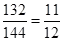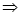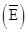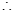# CBSE Solutions for Class 10 Maths

#### Select CBSE Solutions for class 10 Subject & Chapters Wise :

A bag contains 5 blue and 4 purple balls. A ball is drawn at random from the bag. What is the probability of getting a purple ball?

Answer :

4/9

Cards each marked with one of the numbers 4,5,6,…20 are placed in a bag and mixed thoroughly. One card is drawn at random from the bag, what is the probability of getting an even prime number?

Answer :

0

12 defective pencils are accidently mixed with 132 good ones. It is not possible to just look at a pen and tell whether or not it is defective. One pen is taken out at random from this lot. Determine the probability that the pen taken out is a good one.

Answer :

Total number of favourable outcomes = 132 + 12 = 144 Number of favourable outcomes = 132

Hence, P (getting a good pencil) =It is given that in a group of 3 students, the probability of 2 students not having the same birthday is 0.99. What is the probability that the 2 students have the same birthday?

Answer :

Let E be the event of having the same birthdayP(E) = 0.99

But P(E) + P= 1P= 1 P(E) = 1 0.99 = 0.01

If P(E) = 0.04, what is the probability of ‘not E’?

Answer :

Since P(E) + P (not E) = 1P (not E) = 1 – P(E) = 1 – 0.04 = 0.96

The probability of an event is greater than or equal to                         and less than or equal to                           .

Answer :

0,1

The sum of the probabilities of all the elementary events of an experiment is

.

Answer :

1

The probability of an event that is certain to happen is                       . Such an event is called

Answer :

1, Certain Event

The probability of an event that cannot happen is                      . Such an event is called                           .

Answer :

0, impossible event

Probability of event E + Probability of event “not E” =

Answer :

1

One card is drawn from a well-shuffled deck of 52 cards. Find the probability of getting:

(i)a king of black colour

(ii)a face card

(iii)a black face card

(iv)the queen of hearts

(v)a spade

(vi)the queen of diamonds.

Answer :

Each suit contains one king.

Favourable outcomes = 1

Hence, P (a king of black colour) =2/52 =1/26

(ii)There are 12 face cards in a pack.

Favourable outcomes = 12

Hence, P (a face card) =12/52 = 3/13

(iii)There are two suits of black cards, i.e., spade and club. Each suit contains 3 face cards.

Favourable outcomes = 2 x 3 = 6

Hence, P (a black face card) = 6/52 = 3/26

(iv)There are only one queen of heart.

Favourable outcome = 1

Hence, P (the quen of hearts) =1/52

(v)There are 13 cards of spade.

Favourable outcomes = 13

Hence, P (a spade) =13/52 = 1/4

(vi)There is only one queen of diamonds.

Favourable outcome = 1

Hence, P (the queen of diamonds) =1/52

​​​​​Two customers Shyam and Ram are visiting a particular shop in the same week (Tuesday to Saturday). Each is equally likely to visit the shop on any day as on another day. What is the probability that both will visit the shop on

(i) the same day?

(ii) consecutive days?

(iii) different days?

Answer :

Total favourable outcomes associated to the random experiment of visiting a particular shop in the same week (Tuesday to Saturday) by two customers Shyam and Ram are:

(T, T) (T, W) (T, TH) (T, F) (T, S)

(W, T) (W, W) (W, TH) (W, F) (W, S)

(TH, T) (TH, W) (TH, TH) (TH, F) (TH, S)

(F, T) (F, W) (F, TH) (F, F) (F, S)

(S, T) (S, W) (S, TH) (S, F) (S< S)Total number of favourable outcomes = 25

1. The favourable outcomes of visiting on the same day are (T, T), (W, W), (TH, TH), (F, F) and (S, S).Number of favourable outcomes = 5

Hence required probability = 5/25 =1/5

1. The favourable outcomes of visiting on consecutive days are (T, W), (W, T), (W, TH), (TH, W), (TH, F), (F, TH), (S, F) and (F, S).Number of favourable outcomes = 8

Hence required probability =8/25

1. Number of favourable outcomes of visiting on different days are 25 – 5 = 20Number of favourable outcomes = 20

Hence required probability =20/25=4/5

 1 Total outcomes for a dice A 2 2 Total outcomes for 2 dice B 6 3 Total outcomes for a coin C 4 4 Total outcomes for 2 coin D 36

Answer :

1-B, 2-D, 3-A, 4-C

Probability for 2 coins tossed at a time

 1 P(2 heads) A 4 2 P(Total outcomes) B 1/4 3 P(one head & one tail) C 3/4 4 P(atleast one head) D 1/2

Answer :

1-B, 2-A, 3-D, 4-C

Probability for 3 coins tossed at a time

 1 P(2 heads) A 1/2 2 P(atleast 2 tails) B 7/8 3 P(3 heads) C 3/8 4 P(atleast 1 tail) D 1/8

Answer :

1-C, 2-A, 3-D, 4-B

### Take a Test

Choose your Test :

### Chapter 15 : Probability

Probability is one of the chapters in Class 10 maths that contain the concepts that are most likely to be used in daily life. The chapter deals with different topics related to the probability that includes:
1. The difference between experimental probability and theoretical probability 2. Why the probability of a sure event (or certain event) is 1.
3. How the probability of an impossible event is 0?
4. Elementary events.
5. Complementary events.
6. Finding the probability of different events.

### Browse & Download CBSE Books For Class 10 - All Subjects

The GSEB Books for class 10 are designed as per the syllabus followed Gujarat Secondary and Higher Secondary Education Board provides key detailed, and a through solutions to all the questions relating to the GSEB textbooks.

The purpose is to provide help to the students with their homework, preparing for the examinations and personal learning. These books are very helpful for the preparation of examination.

For more details about the GSEB books for Class 10, you can access the PDF which is as in the above given links for the same.